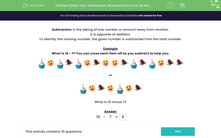Know Your Subtraction: Numbers from 0 to 20 with Pictures (1)

In this worksheet, students practise subtracting numbers within the range 0 to 20.Key stage:  KS 1

Curriculum topic:   Number: Addition and Subtraction

Curriculum subtopic:   Add and Subtract to 20

Difficulty level:Worksheet Overview

Subtraction is the taking of one number or amount away from another.

To identify the missing number, the given number is subtracted from the total number.

Example

What is 16 - 7? You can cross each item off as you subtract to help you.-What is 16 minus 7?